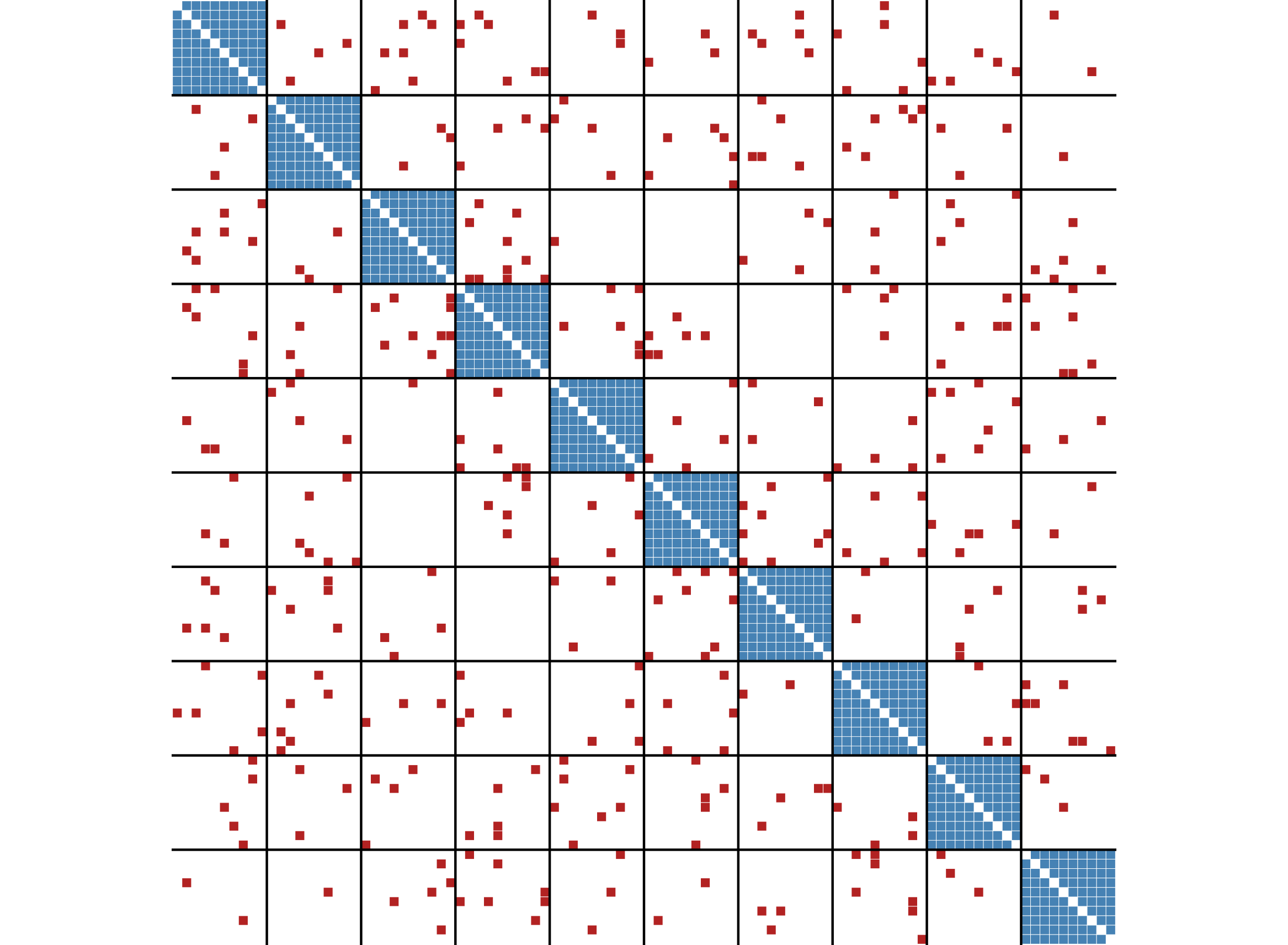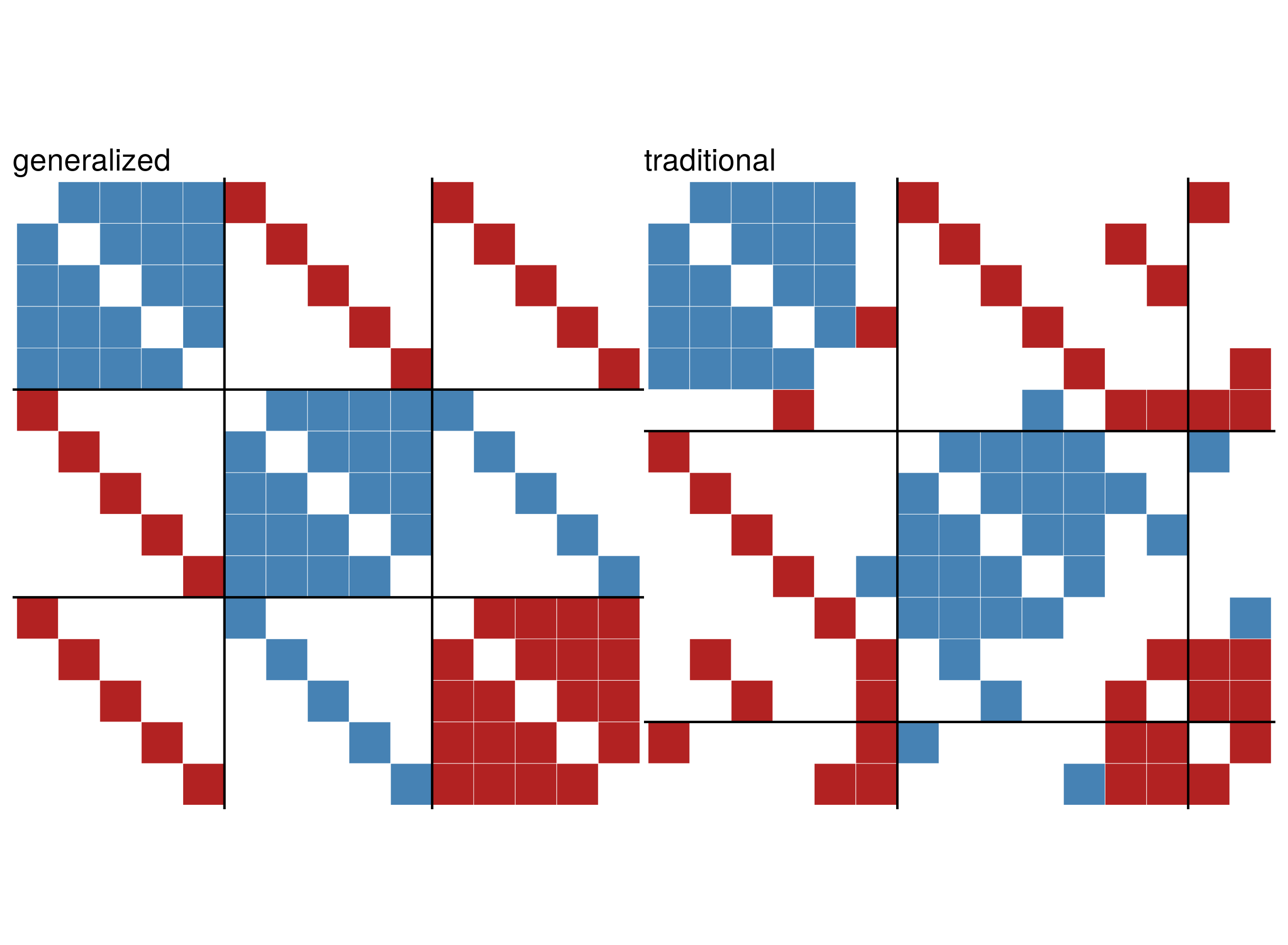Blockmodeling

This vignette describes the two implemented methods for blockmodeling in signed networks.

library(igraph)
library(signnet)

In signed blockmodeling, the goal is to determine k blocks of nodes such that all intra-block edges are positive and inter-block edges are negative. In the example below, we construct a network with a perfect block structure with sample_islands_signed(). The network consists of 10 blocks with 10 vertices each, where each block has a density of 1 (of positive edges). The function signed_blockmodel() is used to construct the blockmodel. The parameter k is the number of desired blocks. alpha is a trade-off parameter. The function minimizes $$P(C)=\alpha N+(1-\alpha)P$$, where $$N$$ is the total number of negative ties within blocks and $$P$$ be the total number of positive ties between blocks.

g <- sample_islands_signed(10,10,1,20)
clu <- signed_blockmodel(g,k = 10,alpha = 0.5)
table(clu$membership) #> #> 1 2 3 4 5 6 7 8 9 10 #> 10 10 10 10 10 10 10 10 10 10 clu$criterion
#>  0

The function returns a list with two entries. The block membership of nodes and the value of $$P(C)$$.

The function ggblock() can be used to plot the outcome of the blockmodel (ggplot2 is required).

ggblock(g,clu$membership,show_blocks = TRUE)If the parameter annealing is set to TRUE, simulated annealing is used in the optimization step. This generally leads to better results but longer runtimes. data("tribes") set.seed(44) #for reproducibility signed_blockmodel(tribes,k = 3,alpha=0.5,annealing = TRUE) #>$membership
#>   1 1 2 2 3 2 2 2 3 3 2 2 3 3 1 1
#>
#> $criterion #>  2 signed_blockmodel(tribes,k = 3,alpha=0.5,annealing = FALSE) #>$membership
#>   1 1 2 2 3 2 2 2 3 3 2 2 3 3 1 1
#>
#> $criterion #>  2 Generalized Blockmodeling The function signed_blockmodel() is only able to provide a blockmodel where the diagonal blocks are positive and off-diagonal blocks are negative. The function signed_blockmodel_general() can be used to specify different block structures. In the below example, we construct a network that contains three blocks. Two have positive and one has negative intra-group ties. The inter-group edges are negative between group one and two, and one and three. Between group two and three, all edges are positive. g1 <- g2 <- g3 <- graph.full(5) V(g1)$name <- as.character(1:5)
V(g2)$name <- as.character(6:10) V(g3)$name <- as.character(11:15)

g <- Reduce("%u%",list(g1,g2,g3))
E(g)$sign <- 1 E(g)$sign[1:10] <- -1

The parameter blockmat is used to specify the desired block structure.

set.seed(424) #for reproducibility
blockmat <- matrix(c(1,-1,-1,-1,1,1,-1,1,-1),3,3,byrow = TRUE)
blockmat
#>      [,1] [,2] [,3]
#> [1,]    1   -1   -1
#> [2,]   -1    1    1
#> [3,]   -1    1   -1

general <- signed_blockmodel_general(g,blockmat,alpha = 0.5)
traditional <- signed_blockmodel(g,k = 3,alpha = 0.5,annealing = TRUE)

c(general$criterion,traditional$criterion)
#>  0 6